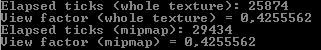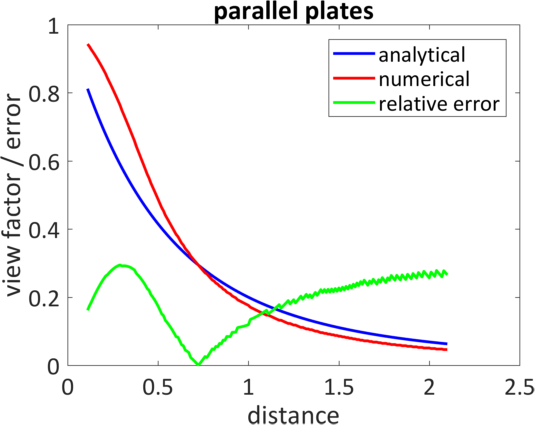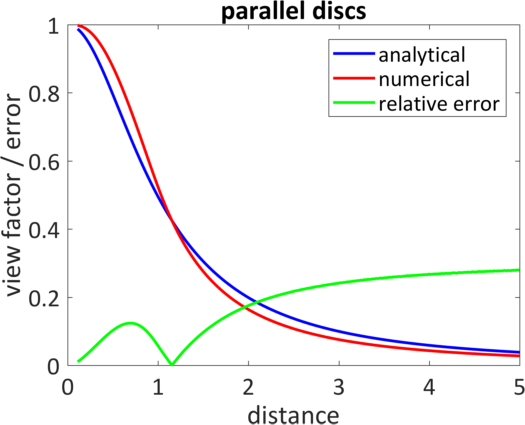Hi @markus,

That’s what I was looking for, thanks. I compared both methods - they yield the same results - and interestingly (but not intuitively), the mipmap solution is slower!?Here is a code snippet for both functions (tex has dimensions of 512x512 pixels).

``````    float GetPixelMean(Texture2D tex)
{
SW.Start();
int N = tex.Width * tex.Height;
float[] PixelValue = new float[N];
tex.GetData(PixelValue);

float Sum = 0;
for (int i = 0; i < PixelValue.Length; i++)
{
Sum += PixelValue[i];
}

long Time = SW.ElapsedTicks;
SW.Stop();
Console.WriteLine("Elapsed ticks: " + Time);

return Sum / N;
}

float GetPixelMeanMipMap(Texture2D tex)
{
SW.Start();
float[] PixelValue = new float;
tex.GetData(tex.LevelCount - 1, null, PixelValue, 0, 1);

long Time = SW.ElapsedTicks;
SW.Stop();
Console.WriteLine("Elapsed ticks: " + Time);

return PixelValue;
}
``````

Maybe the `GetData<>()` call is the bottleneck?

Calling GetData forces the CPU and GPU to synchronize, which can be very costly. See here for more info:
https://msdn.microsoft.com/en-us/library/windows/desktop/bb205132(v=vs.85).aspx#Accessing

Yeah, it does help quite a lot once I scale up the texture size.

Hello @AcidFaucent,

Just for the sake of completeness, I compared the numerical results from the hemicube approach with analytical results in two simple cases:

1. Parallel plates or same dimensions.
2. Parallel discs with radius r and 5r.

The maximum error (green) is somewhere around 30 % and is smallest for view factors between 0.2 and 0.5.It would be interesting to check out how the dual-paraboloid solution compares.

@mgulde sounds about right. Aside from a sampled solution always being off from an analytic one - a hemicube has higher density at the edges that’s going to skew things a great deal (more samples in a band of solid-angles around 45deg outward). Up close you end up with lots of samples in that band and at a distance your samples start to all pile together around the less dense center of the viewing direction.

Just to be sure, you’re only taking the appropriate half of each of the side faces of the cube right?

Yes, I validated it with a couple of sample geometries to get it right.